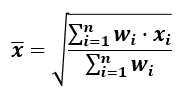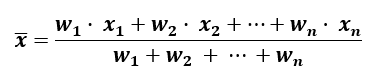# Weighted Average Calculator

Use this weighted mean calculator to calculate the weighted arithmetic average of a set of data. An example use is as a weighted grade calculator. Works easily with copy-paste from a spreadsheet (Excel, etc.).

Share calculator:

Embed this tool:
get code

## What is a weighted average?

A weighted average (a.k.a. weighted mean, weighted arithmetic mean) is a measure of centrality in which some data points contribute differently (more or less) than others. The weighted average is often used when preference needs to be given to certain data points due to their relatively higher importance (e.g. in ranking tasks, indices, etc.) or when there is a need to average statistical measures that come from populations of differing sizes. An example of the former is weighting grades for classes by the number of credits they give or their difficulty (as in weighted GPA). An example of the latter is averaging school grades across a number of school classes with different counts of students in them. A prominent example of using a weigted mean is in finance where the weighted average cost of capital (WACC) is often used to give more weight to financial flows happening sooner versus those happening later in time.

An ordinary average can be seen as a particular case of a weighted average in which all the weights are equal.

The weighted average calculator supports weights expressed on the scale of zero to one (0..1), percentages (0%..100%), ranks, and others (1,3,5, etc.). Note that weight values cannot be negative and while some can be zero, at least one should be positive.

## Weighted average formula

The formula for computing a weighted arithmetic mean is as follows:where xi are values of the variable X, wi are the associated weights, and Σ, the Greek upper-case letter "sigma", is the symbol for the sum operation. This weighted mean equation can be expanded for easier comprehension like so:The formula describes a process in which the values are iteratively multiplied by their weight, added up, and then divided by the sum of weights.

A common example of using weighted averages is to compute a weighted average grade (weighted GPA) from a number of classes or courses taken in high school or university. Assume you have these four classes with the given grades and the number of credits for each class:

Example weighted grade calculation (by number of credits)
Mathematics A 4.0 3
English B 3.0 3
Physics C 2.0 2
Chemistry B+ 3.3 1

In this case the weights are the number of credits for each course. Calculating a weighted GPA based on the number of credits would work like so: (4.0 x 3 + 3.0 x 3 + 2.0 x 2 + 3.3 x 1) / (3 + 3 + 2 + 1) = (12 + 9 + 4 + 3.3) / 9 = 28.3 / 9 = 3.14(4). Compare this to the ordinary mean of 28 / 4 = 3.075.

## Example of a weighted mean score

In this example we will explore the problem of calculating the mean score for laptop offers from different manufacturers. The score will average five components: display quality, battery longevity, weight, CPU performance, and video card performance, each with a different weight. Presented as a table:

Example weights
CharacteristicWeight
Display 5
Battery 4
Weight 3
CPU 3
Video card 4

The above weights represent that the most value is placed on the quality of the display, less on the video card and the battery longevity, and even less on weight and CPU power. Assuming there are five possible choices with roughly equal price and scores on each characteristic on a scale from 1 to 10, the weighted average score for each is calculated in the table below:

Example weighted score comparison
Laptop ID / ScoreDisplayBatteryWeightCPUVideo cardWeighted Score
Laptop #1 6 7 10 9 7 7.53
Laptop #2 9 6 6 9 10 7.42
Laptop #3 8 8 9 6 7 8.11
Laptop #4 7 10 7 9 9 8.11
Laptop #5 7 7 8 7 7 7.15

Scoring several different laptops by using weighted scores calculated using their weighted average allows for easy comparison across all five categories by assigning just a single score for each laptop. Note how #3 and #4 have fairly different scores across the different characteristics yet the same weighted mean score. The same approach can be used when choosing a university, a workplace, a house/apartment, and so on.

#### Cite this calculator & page

If you'd like to cite this online calculator resource and information as provided on the page, you can use the following citation:
Georgiev G.Z., "Weighted Average Calculator", [online] Available at: https://www.gigacalculator.com/calculators/weighted-mean-calculator.php URL [Accessed Date: 01 Apr, 2023].